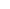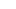Hypothesis Testing | Research Methodology - Research Prospect# Hypothesis Testing

Published by at August 14th, 2021 , Revised On November 5, 2021

## What is a Hypothesis and a Hypothesis Testing?

A hypothesis is considered a belief or assumption that has to be accepted, rejected, proved, or disproved. In contrast, a research hypothesis is a research question for a researcher that has to be proved correct or incorrect through investigation.

Hypothesis testing is a scientific method used for making a decision, drawing conclusions by using a statistical approach. It is used to suggest new ideas by testing theories to know whether or not the sample data support research. A research hypothesis is a predictive statement that has to be tested using scientific methods that join an independent variable to a dependent variable.

Example: The academic performance of student A is better than student B

### Characteristics of the Hypothesis to be Tested

A hypothesis should be:

• Clear and precise
• Capable of being tested
• Able to relate to a variable
• Stated in simple terms
• Consistent with known facts
• Limited in scope and specific
• Tested in a limited timeframe
• Capable of being tested
• Explain the facts in detail

## What is a Null Hypothesis and Alternative Hypothesis?

null hypothesis is a hypothesis when there is no significant relationship between the dependent and the participants’ independent variables

In simple words, it’s a hypothesis that has been put forth but hasn’t been proved as yet. A researcher aims to disprove the theory. The abbreviation “Ho” is used to denote a null hypothesis.

If you want to compare two methods and assume that both methods are equally good, this assumption is considered the null hypothesis.

Example:
In an automobile trial, you feel that the new vehicle’s mileage is similar to the previous model of the car, on average.
You can write it as:
Ho: there is no difference between the mileage of both vehicles.
If your findings don’t support your hypothesis and you get opposite results, this outcome will be considered an alternative hypothesis.

If you assume that one method is better than another method, then it’s considered an alternative hypothesis. The alternative hypothesis is the theory that a researcher seeks to prove and is typically denoted by H1 or HA.

If you support a null hypothesis, it means you’re not supporting the alternative hypothesis. Similarly, if you reject a null hypothesis, it means you are recommending the alternative hypothesis.

Example:
In an automobile trial, you feel that the new vehicle’s mileage is better than the previous model of the vehicle.
You can write it as;
Ha: the two vehicles have different mileage. On average/ the fuel consumption of the new vehicle model is better than the previous model.

If a null hypothesis is rejected during the hypothesis test, even if it’s true, then it is considered as a type-I error. On the other hand, if you don’t dismiss a hypothesis, even if it’s false because you could not identify its falseness, it’s considered a type-II error.

## Hire an Expert Researcher

Orders completed by our expert writers are

• Formally drafted in academic style
• 100% Plagiarism free & 100% Confidential
• Never resold
• Include unlimited free revisions
• Completed to match exact client requirements## How to Conduct Hypothesis Testing?

### Step 1: State the Null and Alternative Hypothesis

Once you develop a research hypothesis, it’s important to state it is as a Null hypothesis (Ho) and an Alternative hypothesis (Ha) to test it statistically.

A null hypothesis is a preferred choice as it provides the opportunity to test the theory. In contrast, you can accept the alternative hypothesis when the null hypothesis has been rejected.

Example:
You want to identify a relationship between obesity of men and women and the modern living style. You develop a hypothesis that women, on average, gain weight quickly compared to men.
Then you write it as:
Ho: Women, on average, don’t gain weight quickly compared to men.
Ha: Women, on average, gain weight quickly compared to men.

### Step 2: Data Collection

Hypothesis testing follows the statistical method, and statistics are all about data. It’s challenging to gather complete information about a specific population you want to study. You need to gather the data obtained through a large number of samples from a specific population.

Example:
Suppose you want to test the difference in the rate of obesity between men and women. You should include an equal number of men and women in your sample. Then investigate various aspects such as their lifestyle, eating patterns and profession, and any other variables that may influence average weight.
You should also determine your study’s scope, whether it applies to a specific group of population or worldwide population. You can use available information from various places, countries, and regions.

### Step 3: Select Appropriate Statistical Test

There are many types of statistical tests, but we discuss the most two common types below, such as One-sided and two-sided tests.

Note: Your choice of the type of test depends on the purpose of your study

#### One-sided Test

In the one-sided test, the values of rejecting a null hypothesis are located in one tail of the probability distribution. The set of values is less or higher than the critical value of the test. It is also called a one-tailed test of significance.

Example:
If you want to test that all mangoes in a basket are ripe.
You can write it as:
Ho: All mangoes in the basket, on average, are ripe.
If you find all ripe mangoes in the basket, the null hypothesis you developed will be true.

#### Two-sided Test

In the two-sided test, the values of rejecting a null hypothesis are located on both tails of the probability distribution. The set of values is less or higher than the first critical value of the test and higher than the second critical value test. It is also called a two-tailed test of significance.

Example:
Nothing can be explicitly said whether all mangoes are ripe in the basket.
If you reject the null hypothesis (Ho: All mangoes in the basket, on average, are ripe), then it means all mangoes in the basket are not likely to be ripe. A few mangoes could be raw as well.

## Get statistical analysis help at an affordable price

We have:

• An expert statistician will complete your work
• Rigorous quality checks
• Confidentiality and reliability
• Any statistical software of your choice
• Free Plagiarism Report### Step 4: Select the Level of Significance

When you reject a null hypothesis, even if it’s true during a statistical hypothesis, it is considered the significance level. It is the probability of a type one error. The significance should be as minimum as possible to avoid the type-I error, which is considered severe and should be avoided.

If the significance level is minimum, then it prevents the researchers from false claims.

The significance level is denoted by P, and it has given the value of 0.05 (P=0.05)

If the P-Value is less than 0.05, then the difference will be significant. If the P-value is higher than 0.05, then the difference is non-significant.

Example:
Suppose you apply a one-sided test to test whether women gain weight quickly compared to men. You get to know about the average weight between men and women and the factors promoting weight gain.

### Step 5: Find out Whether the Null Hypothesis is Rejected or Supported

After conducting a statistical test, you should identify whether your null hypothesis is rejected or accepted based on the test results. It would help if you observed the P-value for this.

Example:
If you find the P-value of your test is less than 0.5/5%, then you need to reject your null hypothesis (Ho: Women, on average, don’t gain weight quickly compared to men).
On the other hand, if a null hypothesis is rejected, then it means the alternative hypothesis might be true (Ha: Women, on average, gain weight quickly compared to men. If you find your test’s P-value is above 0.5/5%, then it means your null hypothesis is true.

### Step 6: Present the Outcomes of Your Study

The final step is to present the outcomes of your study. You need to ensure whether you have met the objectives of your research or not.

In the discussion section and conclusion, you can present your findings by using supporting evidence and conclude whether your null hypothesis was rejected or supported.

In the result section, you can summarize your study’s outcomes, including the average difference and P-value of the two groups.

If we talk about the findings, our study your results will be as follows:

Example:
In the study of identifying whether women gain weight quickly compared to men, we found the P-value is less than 0.5.
Hence, we can reject the null hypothesis (Ho: Women, on average, don’t gain weight quickly than men) and conclude that women may likely gain weight quickly than men.

Did you know in your academic paper you should not mention whether you have accepted or rejected the null hypothesis?

Always remember that you either conclude to reject Ho in favor of Haor do not reject Ho. It would help if you never rejected Ha or even accept Ha.

Suppose your null hypothesis is rejected in the hypothesis testing. If you conclude reject Ho in favor of Haor do not reject Ho, then it doesn’t mean that the null hypothesis is true. It only means that there is a lack of evidence against Ho in favour of Ha. If your null hypothesis is not true, then the alternative hypothesis is likely to be true.

Example:
We found that the P-value is less than 0.5. Hence, we can conclude reject Ho in favour of Ha (Ho: Women, on average, don’t gain weight quickly than men) reject Ho in favour of Ha. However, rejected in favour of Ha means (Ha: women may likely to gain weight quickly than men)Troy has been the leading content creator for Research Prospect since 2017. He loves to write about the different types of data collection and data analysis methods used in research. Troy has also been lucky enough to work as an editor for BBC.